# Long Transmission Line: What is it? (Plus ABCD Parameters)

## What is Long Transmission Line?

A long transmission line is defined as a transmission line with an effective length more than 250 km (150 miles). Unlike short transmission lines and medium transmission lines, it is no longer reasonable to assume that the line parameters are lumped. To accurately model a long transmission line we must consider the exact effect of the distributed parameters over the entire length of the line. Although this makes the calculation of ABCD parameters of transmission line more complex, it also allows us to derive expressions for the voltage and current at any point along the line.In a long transmission line the line constants are uniformly distributed over the entire length of line. This is because the effective circuit length is much higher than what it was for the former models (long and medium line) and hence we can no longer make the following approximations:

1. Ignoring the shunt admittance of the network, like in a small transmission line model.
2. Considering the circuit impedance and admittance to be lumped and concentrated at a point as was the case for the medium line model.

Rather, for all practical reasons, we should consider the circuit impedance and admittance being distributed over the entire circuit length as shown in the figure below. The calculations of circuit parameters, for this reason, are going to be slightly more rigorous as we will see here. For accurate modelling to determine circuit parameters let us consider the circuit of the long transmission line as shown in the diagram below.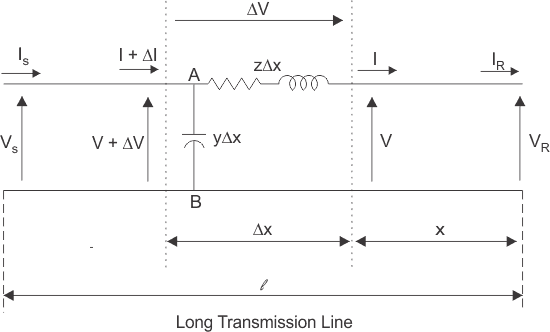Here a line of length l > 250km is supplied with a sending end voltage and current of VS and IS respectively, whereas the VR and IR are the values of voltage and current obtained from the receiving end. Lets us now consider an element of infinitely small length Δx at a distance x from the receiving end as shown in the figure where.
V = value of voltage just before entering the element Δx.
I = value of current just before entering the element Δx.
V+ΔV = voltage leaving the element Δx.
I+ΔI = current leaving the element Δx.
ΔV = voltage drop across element Δx.
zΔx = series impedance of element Δx
yΔx = shunt admittance of element Δx
Where, Z = z l and Y = y l are the values of total impedance and admittance of the long transmission line.

Therefore, the voltage drop across the infinitely small element Δx is given by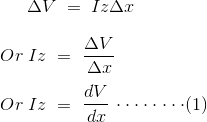Now to determine the current ΔI, we apply KCL to node A.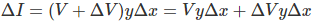Since the term ΔV yΔx is the product of 2 infinitely small values, we can ignore it for the sake of easier calculation.
Therefore, we can write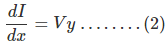Now derivating both sides of eq (1) w.r.t x,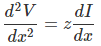Now substitutingfrom equation (2)The solution of the above second order differential equation is given by.Derivating equation (4) w.r.to x.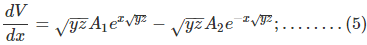Now comparing equation (1) with equation (5)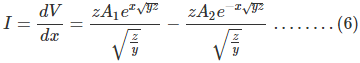Now to go further let us define the characteristic impedance Zc and propagation constant δ of a long transmission line asThen the voltage and current equation can be expressed in terms of characteristic impedance and propagation constant asNow at x=0, V= VR and I= Ir. Substituting these conditions to equation (7) and (8) respectively.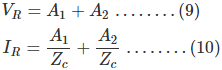Solving equation (9) and (10),
We get values of A1 and A2 as,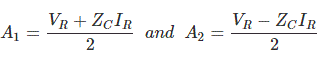Now applying another extreme condition at x = l, we have V = VS and I = IS.
Now to determine VS and IS we substitute x by l and put the values of A1 and
A2 in equation (7) and (8) we get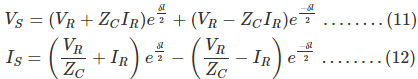By trigonometric and exponential operators we know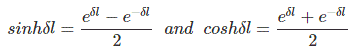Therefore, equation (11) and (12) can be re-written as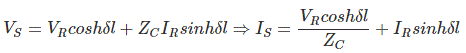Thus compared with the general circuit parameters equation, we get the ABCD parameters of a long transmission line as,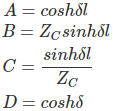Want To Learn Faster? 🎓
Get electrical articles delivered to your inbox every week.
No credit card required—it’s 100% free.